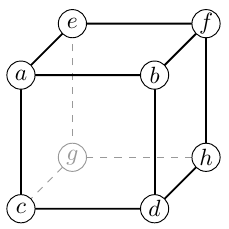# Announcing the Summer 2020 Algorithm Competition and Prizes

 This competition ended. The results are available here: https://github.com/PulledPork0/AlgoCompetition/tree/master/resultsThis algorithm competition allows programmers and math puzzle enthusiasts to compete by writing an algorithm in python to solve a math puzzle using as little "computational cost" as possible.

A python utility is supplied which allows anyone to easily test their algorithm to verify its correctness as well as have its computational cost calculated with some example inputs.

The contest ends on August 31, 2020. The submissions will then be officially tested and the entry which uses the least amount of computational cost to solve some pre-selected instances of the puzzle will be awarded the grand prize (see constest rules for details).

The current prize pool is 2 ETH (approximately \$450 USD).

## The main puzzle

This contest’s puzzle is inspired by part of a cryptographic operation used by Chia in a 'proof of time' for their cryptocoin. This is a fascinating subject which you can read more about on their site. However, this contest will not require any knowledge on such proofs.

The contest problem can be approached with basic algebra skills supplemented with knowledge of how to solve linear equations involving integers.

What follows is an algebra description of the problem, which skips the deeper math. For those wanting to explore the details themselves, there is a short introduction in this document and a reference section at the end with links to some helpful material.

### The puzzle setup

Consider a cube with integers on each corner, and the values labelled .

Associated with these 8 cube values are three tuples of values , , , given by what will be referred to as the "cube equations":

Given any cube values , it is clear that the tuple values , etc. are uniquely determined.

What is not obvious from this, is that given any tuple values if there is a solution, the cube values are uniquely deteremined up to an overall sign. (A sketch of a proof is provided in 'Useful algebra results'.)

So up to an overall sign, a cube can be uniquely referred to by either the eight corner values or the three tuples.

### Algorithm to create

In the competition, the goal is to write a python function which will take as input specifying a cube, with the following additional guarantees that may make the algebra easier:

• the first two tuples are equal

• , where is a prime and .

The function must then calculate and return new cube values such that

with as little computational cost as possible.

This function will be tested by giving it an initial cube and then repeated application of the function on its own output. So it is also advantageous to keep the size of the integers in your cube solution from getting larger each time the function is called.

(As discussed in the 'Skim Some Deeper Math' section, the in the above equation is referring to an equivalence relation that gives more freedom in the solution if desired. The details of this freedom is shown explicitly in 'Equivalence tranformations'. While this extra freedom may be helpful, pursing those details is not strictly required, and replacing the with a normal in that equation would still give acceptible solutions.)